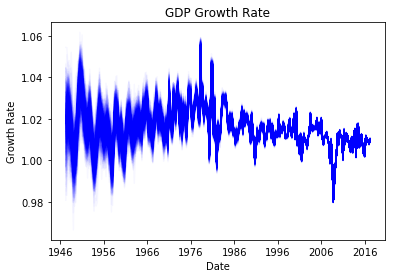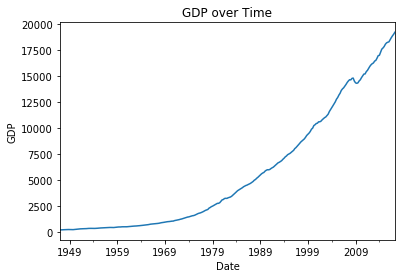### Bayesian Time Varying Coefficients in PYMC3Okay so today I want to talk about something really cool that you can do with time-series / panel data. That is, you can allow the coefficients in the model change over time. It is a really neat idea, but it creates a challenge for writing down the model in most of the traditional regression packages in python. Often it requires the specification of a custom likelihood function. That is fine, but if you want to go Bayesian, then PYMC3 has your back. The fine people who developed this library have made it easy for you to build and estimate models with time varying coefficients.

So why is this important? If you have read my blog posts about ARIMA type models. (Get to them from these links: ARIMA models, SARIMA models, and ARIMAX models) Then you already know that the goal of these models is to get you back to Stationarity. Or rather you need a stationary model in order for these models to do their job. So what is the purpose of time-varying coefficients? They also help you deal with non-stationarity of your data. Essentially, you are making the assumption that it is not the data itself that is non-stationary, rather, it is the data generating process that is non-stationary.

This approach is appealing for at least two reasons. First, the data is viewed as the result of a data generating process, the non-stationary nature of the data is a consequence of the evolving model, not an inherent feature in the data. Second, the model is going to be very flexible and likely to fit the data very well.

So the first thing that we’re going to need is some data. As a person with a PhD in economics, GDP of the United States of America sounds like it could be a fun series to work with. You can obtain this data thanks to the St. Louis branch of the federal reserve here This will give you quarterly data for U.S. GDP all the way back to 1947. This time series is actually pretty well behaved it is non-stationary though. But we’ll push forward anyway.

### Bring on the Code

We can start by pulling in the libraries that we need, and getting the raw data into python.

import pandas as pd
import pymc3 as pm
import matplotlib.pyplot as plt



Alright, now that we have the data in python. Let’s go right ahead and take a look at the dataset just to see what it looks like.

plt.plot(df['DATE'],df['GDP'])
plt.title('GDP over Time')
plt.xlabel('Date')
plt.ylabel('GDP')
plt.show()


Which gives you a nice looking, almost exponential, growth curve. In fact, other than the dip in the late 2000s it is hard to see the other recessions. They are there, and the growth rate of GDP isn’t constant across time.Growth in GDP

So we can see the growth. What our goal with the model, is to build a model of the growth rates of GDP and see how the growth rates change over time. Now of course, we could simply compute the growth rates, and plot them. That would be simple enough to do, and later on we will compare this method to the results from the model.

df['lag'] = df['GDP'].shift()
df.dropna(inplace=True)
with pm.Model() as model:
sigma = pm.Exponential('sigma', 1./.02, testval=.1)
nu = pm.Exponential('nu', 1./10)
beta = pm.GaussianRandomWalk('beta',sigma**-2,shape=len(df['GDP']))
observed = pm.Normal('observed', mu=beta*df['lag'], sd = 1/nu, observed = df['GDP'])

trace = pm.sample()


So what does this model do? Other than some noise parameters sigma, which controls the step size on the random walk for the beta coefficient, and nu, which controls the width of the likelihood function, we have one more coefficient beta, which measures the growth of GDP. The way that we accomplish this is we take the previous period’s GDP and let it grow by beta. If beta is greater than 1 GDP will be bigger in the next period if it is less than 1 GDP will shrink.

After training the model, which on my ancient laptop took less than five minutes, we can visualize how the growth rate in GDP changed over time. Now, notice that we have a full posterior distribution on the growth rates for every quarter. We can visualize this distribution by plotting the trace over time.

plt.plot(df.index,trace['beta'].T, 'b', alpha=.03)
plt.title('GDP Growth Rate')
plt.xlabel('Date')
plt.ylabel('Growth Rate')
plt.show()


Running this snippet of code will yield this graph:Posterior of Growth Rates

That’s pretty good. The only thing is that we can’t really tell how good of a fit we have achieved. So think back to when I said that this was a silly way to calculate growth rates. We could simply calculate the growth rates directly. You can do this using the following mathematical operation:$g=log(x_{t})-log(x_{t-1})$

Where$g$ is the growth rate,$log$ is the natural logarithm, and$x_{t}$ is GDP at time t. This will give the percentage change. It isn’t perfectly the same thing that we calculated with the model. The model technically gave us quarterly growth factors. To transform these growth rates into the comparable growth factors we calculated with the model, you just have to add 1. We do this, and add a plot of the calculated growth rates to the model. You can think of these growth rates as the ground truth for our model. Here is the code:

plt.plot(df.index,trace['beta'].T, 'b', alpha=.03)
plt.plot(df.index,1+(np.log(df['GDP'])-np.log(df['lag'])),'r',label='True Growth Rate')
plt.title('GDP Growth Rate')
plt.legend()
plt.xlabel('Date')
plt.ylabel('Growth Rate')
plt.show()


Which yields the following graph:Model output with ground truth

A couple of economics nerdy things. Notice that the distribution was wider for observations before the late 70s and early 80s and then it stabilized. This roughly corresponds to the time that Paul Volcker was chairman of the Federal Reserve. So it seems like his efforts to stabilize the economy paid off. Second you can clearly see the negative growth of the recession of 2007 and 2008. It actually, wasn’t all that bad if you look at the late 40s and early 50s. The only difference was how volatile that period was. Those losses were almost immeadiately followed by a period of equal expansion, The recession in 2007 and 2008 (which I can’t believe are already 10 years behind us!) did not have such a massive uptick following it. So that’s why we don’t remember those down swings in the late 40s and early 50s but 10 years ago we had a tough time.

### Full Code

import pandas as pd
import pymc3 as pm
import matplotlib.pyplot as plt

df.index = pd.to_datetime(df['DATE'])

df['GDP'].plot()
plt.title('GDP over Time')
plt.xlabel('Date')
plt.ylabel('GDP')
plt.show()

df['lag'] = df['GDP'].shift()
df.dropna(inplace=True)
with pm.Model() as model:
sigma = pm.Exponential('sigma', 1./.02, testval=.1)
nu = pm.Exponential('nu', 1./10)
beta = pm.GaussianRandomWalk('beta',sigma**-2,shape=len(df['GDP']))
observed = pm.Normal('observed', mu=beta*df['lag'], sd = 1/nu, observed = df['GDP'])

trace = pm.sample()

plt.plot(df.index,trace['beta'].T, 'b', alpha=.03)
plt.title('GDP Growth Rate')
plt.xlabel('Date')
plt.ylabel('Growth Rate')
plt.show()

plt.plot(df.index,trace['beta'].T, 'b', alpha=.03)
plt.plot(df.index,1+(np.log(df['GDP'])-np.log(df['lag'])),'r',label='True Growth Rate')
plt.title('GDP Growth Rate')
plt.legend()
plt.xlabel('Date')
plt.ylabel('Growth Rate')
plt.show()